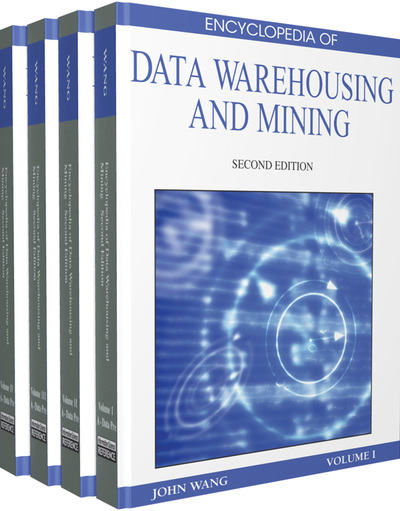# Modeling Quantiles

Claudia Perlich (IBM T.J. Watson Research, USA), Saharon Rosset (IBM T.J. Watson Research, USA) and Bianca Zadrozny (Universidade Federal Fluminense, Brazil)
DOI: 10.4018/978-1-60566-010-3.ch205
Available
\$37.50
No Current Special Offers

## Abstract

One standard Data Mining setting is defines by a set of n observations on a variable of interest Y and a set of p explanatory variables, or features, x = (x1,...,xp), with the objective of finding a ‘dependence’ of Y on x. Such dependencies can either be of direct interest by themselves or used in the future to predict a Y given an observed x. This typically leads to a model for a conditional central tendency of Y|x, usually the mean E(Y|x). For example, under appropriate model assumptions, Data Mining based on a least squares loss function (like linear least squares or most regression tree approaches), is as a maximum likelihood approach to estimating the conditional mean. This chapter considers situations when the value of interest is not the conditional mean of a continuous variable, but rather a different property of the conditional distribution P(Y|x), in particular a specific quantile of this distribution. Consider for instance the 0.9th quantile of P(Y|x), which is the function c(x) such that P(Y
Chapter Preview
Top

## Introduction

One standard Data Mining setting is defines by a set of n observations on a variable of interest Y and a set of p explanatory variables, or features, x = (x1,...,xp), with the objective of finding a ‘dependence’ of Y on x. Such dependencies can either be of direct interest by themselves or used in the future to predict a Y given an observed x. This typically leads to a model for a conditional central tendency of Y|x, usually the mean E(Y|x). For example, under appropriate model assumptions, Data Mining based on a least squares loss function (like linear least squares or most regression tree approaches), is as a maximum likelihood approach to estimating the conditional mean.

This chapter considers situations when the value of interest is not the conditional mean of a continuous variable, but rather a different property of the conditional distribution P(Y|x), in particular a specific quantile of this distribution. Consider for instance the 0.9th quantile of P(Y|x), which is the function c(x) such that P(Y<c(x)|x) = 0.9. As discussed in the main section, these problems (of estimating conditional mean vs. conditional high quantile) may be equivalent under simplistic assumptions about our models, but in practice they are usually not. We are typically interested in modeling extreme quantiles because they represent a desired ‘prediction’ in many business and scientific domains. Consider for example the motivating Data Mining task of estimating customer wallets from existing customer transaction data, which is of great practical interest for marketing and sales. A customer’s wallet for a specific product category is the total amount this customer can spend in this product category. The vendor observes what the customers actually bought from him in the past, but does not typically have access to the customer’s budget allocation decisions, their spending with competitors, etc. Information about customer’s wallet, as an indicator of their potential for growth, is considered extremely valuable for marketing, resource planning and other tasks. For a detailed survey of the motivation, problem definition, see Rosset et al. 2005. In that paper we propose the definition of a customer’s REALISTIC wallet as the 0.9th or 0.95th quantile of their conditional spending - this can be interpreted as the quantity that they may spend in the best case scenario. This task of modeling what a vendor can hope for rather than could expect turns out to be of great interest in multiple other business domains, including:

• When modeling sales prices of houses, cars or any other product, the seller may be very interested in the price they may aspire to get for their asset if they are successful in negotiations. This is clearly different from the ‘average’ price for this asset and is more in line with a high quantile of the price distribution of equivalent assets. Similarly, the buyer may be interested in the symmetric problem of modeling a low quantile.

• In outlier and fraud detection applications we may often have a specific variable (such as total amount spent on a credit card) whose degree of ‘outlyingness’ we want to examine for each one of a set of customers or observations. This degree can often be well approximated by the quantile of the conditional spending distribution given the customer’s attributes. For identifying outliers we may just want to compare the actual spending to an appropriate high quantile, say 0.95.

• The opposite problem of the same notion of ‘how bad can it get’ is a very relevant component of financial modeling and in particular Value-at-Risk (Chernozhukov and Umantsev, 2001).

Addressing this task of quantile predictions, various researches have proposed methods that are often adaptations of standard expected value modeling approaches to the quantile modeling problem, and demonstrated that their predictions are meaningfully different from traditional expected value models.

## Complete Chapter List

Search this Book:
Reset